Related Articles
Doubly Linked List | Set 1 (Introduction and Insertion)
• Difficulty Level : Easy
• Last Updated : 09 Mar, 2021

We strongly recommend to refer following post as a prerequisite of this post.
Linked List Introduction
Inserting a node in Singly Linked List
A Doubly Linked List (DLL) contains an extra pointer, typically called previous pointer, together with next pointer and data which are there in singly linked list.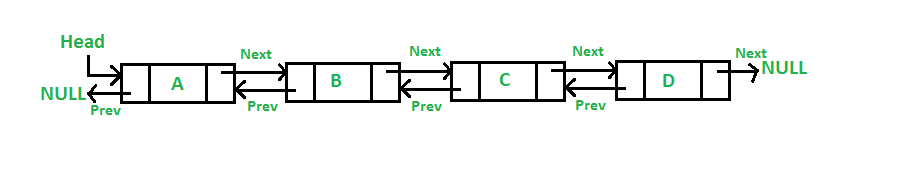Following is representation of a DLL node in C language.

## C

 `/* Node of a doubly linked list */``struct` `Node {``    ``int` `data;``    ``struct` `Node* next; ``// Pointer to next node in DLL``    ``struct` `Node* prev; ``// Pointer to previous node in DLL``};`

## Java

 `// Class for Doubly Linked List``public` `class` `DLL {``    ``Node head; ``// head of list` `    ``/* Doubly Linked list Node*/``    ``class` `Node {``        ``int` `data;``        ``Node prev;``        ``Node next;` `        ``// Constructor to create a new node``        ``// next and prev is by default initialized as null``        ``Node(``int` `d) { data = d; }``    ``}``}`

## Python3

 `# Node of a doubly linked list``class` `Node:``    ``def` `__init__(``self``, ``next``=``None``, prev``=``None``, data``=``None``):``        ``self``.``next` `=` `next` `# reference to next node in DLL``        ``self``.prev ``=` `prev ``# reference to previous node in DLL``        ``self``.data ``=` `data`

Following are advantages/disadvantages of doubly linked list over singly linked list.
Advantages over singly linked list
1) A DLL can be traversed in both forward and backward direction.
2) The delete operation in DLL is more efficient if pointer to the node to be deleted is given.
3) We can quickly insert a new node before a given node.
In singly linked list, to delete a node, pointer to the previous node is needed. To get this previous node, sometimes the list is traversed. In DLL, we can get the previous node using previous pointer.

Disadvantages over singly linked list
1) Every node of DLL Require extra space for an previous pointer. It is possible to implement DLL with single pointer though (See this and this).
2) All operations require an extra pointer previous to be maintained. For example, in insertion, we need to modify previous pointers together with next pointers. For example in following functions for insertions at different positions, we need 1 or 2 extra steps to set previous pointer.
Insertion
A node can be added in four ways
1) At the front of the DLL
2) After a given node.
3) At the end of the DLL
4) Before a given node.

1) Add a node at the front: (A 5 steps process)
The new node is always added before the head of the given Linked List. And newly added node becomes the new head of DLL. For example if the given Linked List is 10152025 and we add an item 5 at the front, then the Linked List becomes 510152025. Let us call the function that adds at the front of the list is push(). The push() must receive a pointer to the head pointer, because push must change the head pointer to point to the new node (See this)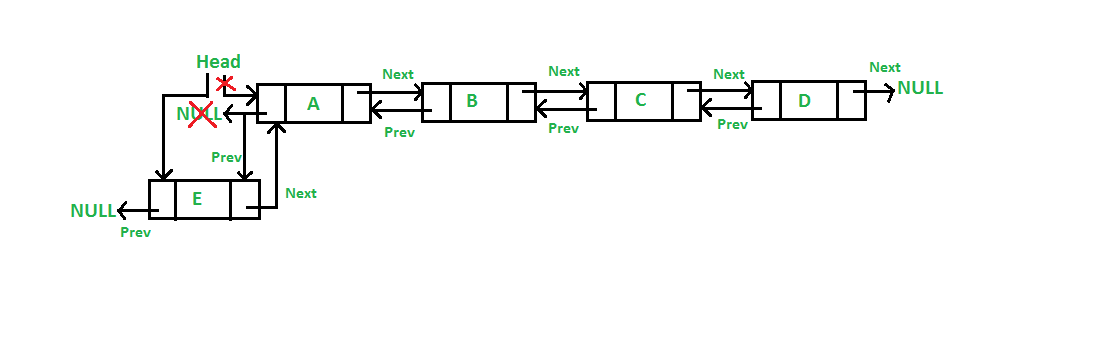Following are the 5 steps to add node at the front.

## C

 `/* Given a reference (pointer to pointer) to the head of a list``   ``and an int, inserts a new node on the front of the list. */``void` `push(``struct` `Node** head_ref, ``int` `new_data)``{``    ``/* 1. allocate node */``    ``struct` `Node* new_node = (``struct` `Node*)``malloc``(``sizeof``(``struct` `Node));` `    ``/* 2. put in the data  */``    ``new_node->data = new_data;` `    ``/* 3. Make next of new node as head and previous as NULL */``    ``new_node->next = (*head_ref);``    ``new_node->prev = NULL;` `    ``/* 4. change prev of head node to new node */``    ``if` `((*head_ref) != NULL)``        ``(*head_ref)->prev = new_node;` `    ``/* 5. move the head to point to the new node */``    ``(*head_ref) = new_node;``}`

## Java

 `// Adding a node at the front of the list``public` `void` `push(``int` `new_data)``{``    ``/* 1. allocate node``    ``* 2. put in the data */``    ``Node new_Node = ``new` `Node(new_data);` `    ``/* 3. Make next of new node as head and previous as NULL */``    ``new_Node.next = head;``    ``new_Node.prev = ``null``;` `    ``/* 4. change prev of head node to new node */``    ``if` `(head != ``null``)``        ``head.prev = new_Node;` `    ``/* 5. move the head to point to the new node */``    ``head = new_Node;``}`

## Python3

 `# Adding a node at the front of the list``def` `push(``self``, new_data):` `    ``# 1 & 2: Allocate the Node & Put in the data``    ``new_node ``=` `Node(data ``=` `new_data)` `    ``# 3. Make next of new node as head and previous as NULL``    ``new_node.``next` `=` `self``.head``    ``new_node.prev ``=` `None` `    ``# 4. change prev of head node to new node``    ``if` `self``.head ``is` `not` `None``:``        ``self``.head.prev ``=` `new_node` `    ``# 5. move the head to point to the new node``    ``self``.head ``=` `new_node` `# This code is contributed by jatinreaper`

## C#

 `// Adding a node at the front of the list``public` `void` `push(``int` `new_data)``{``  ` `    ``/* 1. allocate node``    ``* 2. put in the data */``    ``Node new_Node = ``new` `Node(new_data);` `    ``/* 3. Make next of new node as head and previous as NULL */``    ``new_Node.next = head;``    ``new_Node.prev = ``null``;` `    ``/* 4. change prev of head node to new node */``    ``if` `(head != ``null``)``        ``head.prev = new_Node;` `    ``/* 5. move the head to point to the new node */``    ``head = new_Node;``}` `// This code is contributed by aashish1995`

Four steps of the above five steps are same as the 4 steps used for inserting at the front in singly linked list. The only extra step is to change previous of head.
2) Add a node after a given node.: (A 7 steps process)
We are given pointer to a node as prev_node, and the new node is inserted after the given node.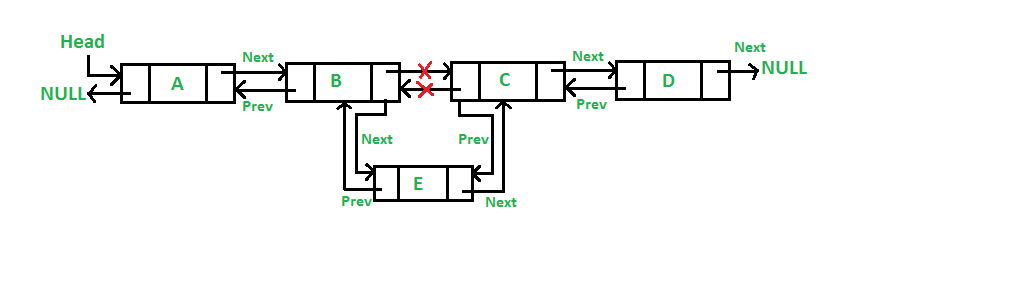## C

 `/* Given a node as prev_node, insert a new node after the given node */``void` `insertAfter(``struct` `Node* prev_node, ``int` `new_data)``{``    ``/*1. check if the given prev_node is NULL */``    ``if` `(prev_node == NULL) {``        ``printf``(``"the given previous node cannot be NULL"``);``        ``return``;``    ``}` `    ``/* 2. allocate new node */``    ``struct` `Node* new_node = (``struct` `Node*)``malloc``(``sizeof``(``struct` `Node));` `    ``/* 3. put in the data  */``    ``new_node->data = new_data;` `    ``/* 4. Make next of new node as next of prev_node */``    ``new_node->next = prev_node->next;` `    ``/* 5. Make the next of prev_node as new_node */``    ``prev_node->next = new_node;` `    ``/* 6. Make prev_node as previous of new_node */``    ``new_node->prev = prev_node;` `    ``/* 7. Change previous of new_node's next node */``    ``if` `(new_node->next != NULL)``        ``new_node->next->prev = new_node;``}`

## Java

 `/* Given a node as prev_node, insert a new node after the given node */``public` `void` `InsertAfter(Node prev_Node, ``int` `new_data)``{` `    ``/*1. check if the given prev_node is NULL */``    ``if` `(prev_Node == ``null``) {``        ``System.out.println(``"The given previous node cannot be NULL "``);``        ``return``;``    ``}` `    ``/* 2. allocate node``    ``* 3. put in the data */``    ``Node new_node = ``new` `Node(new_data);` `    ``/* 4. Make next of new node as next of prev_node */``    ``new_node.next = prev_Node.next;` `    ``/* 5. Make the next of prev_node as new_node */``    ``prev_Node.next = new_node;` `    ``/* 6. Make prev_node as previous of new_node */``    ``new_node.prev = prev_Node;` `    ``/* 7. Change previous of new_node's next node */``    ``if` `(new_node.next != ``null``)``        ``new_node.next.prev = new_node;``}`

## Python3

 `# Given a node as prev_node, insert``# a new node after the given node` `def` `insertAfter(``self``, prev_node, new_data):` `        ``# 1. check if the given prev_node is NULL``        ``if` `prev_node ``is` `None``:``            ``print``(``"This node doesn't exist in DLL"``)``            ``return` `        ``#2. allocate node  & 3. put in the data``        ``new_node ``=` `Node(data ``=` `new_data)` `        ``# 4. Make next of new node as next of prev_node``        ``new_node.``next` `=` `prev_node.``next` `        ``# 5. Make the next of prev_node as new_node``        ``prev_node.``next` `=` `new_node` `        ``# 6. Make prev_node as previous of new_node``        ``new_node.prev ``=` `prev_node` `        ``# 7. Change previous of new_node's next node */``        ``if` `new_node.``next` `is` `not` `None``:``            ``new_node.``next``.prev ``=` `new_node` `#  This code is contributed by jatinreaper`

## C#

 `/* Given a node as prev_node, insert a new node after the given node */``public` `void` `InsertAfter(Node prev_Node, ``int` `new_data)``{` `    ``/*1. check if the given prev_node is NULL */``    ``if` `(prev_Node == ``null``) {``        ``Console.WriteLine(``"The given previous node cannot be NULL "``);``        ``return``;``    ``}` `    ``/* 2. allocate node``    ``* 3. put in the data */``    ``Node new_node = ``new` `Node(new_data);` `    ``/* 4. Make next of new node as next of prev_node */``    ``new_node.next = prev_Node.next;` `    ``/* 5. Make the next of prev_node as new_node */``    ``prev_Node.next = new_node;` `    ``/* 6. Make prev_node as previous of new_node */``    ``new_node.prev = prev_Node;` `    ``/* 7. Change previous of new_node's next node */``    ``if` `(new_node.next != ``null``)``        ``new_node.next.prev = new_node;``}` `// This code is contributed by aashish1995`

Five of the above steps step process are same as the 5 steps used for inserting after a given node in singly linked list. The two extra steps are needed to change previous pointer of new node and previous pointer of new node’s next node.
3) Add a node at the end: (7 steps process)
The new node is always added after the last node of the given Linked List. For example if the given DLL is 510152025 and we add an item 30 at the end, then the DLL becomes 51015202530.
Since a Linked List is typically represented by the head of it, we have to traverse the list till end and then change the next of last node to new node.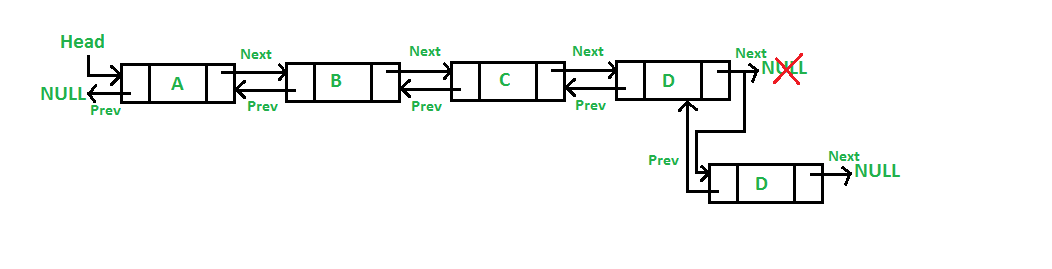Following are the 7 steps to add node at the end.

## C

 `/* Given a reference (pointer to pointer) to the head``   ``of a DLL and an int, appends a new node at the end  */``void` `append(``struct` `Node** head_ref, ``int` `new_data)``{``    ``/* 1. allocate node */``    ``struct` `Node* new_node = (``struct` `Node*)``malloc``(``sizeof``(``struct` `Node));` `    ``struct` `Node* last = *head_ref; ``/* used in step 5*/` `    ``/* 2. put in the data  */``    ``new_node->data = new_data;` `    ``/* 3. This new node is going to be the last node, so``          ``make next of it as NULL*/``    ``new_node->next = NULL;` `    ``/* 4. If the Linked List is empty, then make the new``          ``node as head */``    ``if` `(*head_ref == NULL) {``        ``new_node->prev = NULL;``        ``*head_ref = new_node;``        ``return``;``    ``}` `    ``/* 5. Else traverse till the last node */``    ``while` `(last->next != NULL)``        ``last = last->next;` `    ``/* 6. Change the next of last node */``    ``last->next = new_node;` `    ``/* 7. Make last node as previous of new node */``    ``new_node->prev = last;` `    ``return``;``}`

## Java

 `// Add a node at the end of the list``void` `append(``int` `new_data)``{``    ``/* 1. allocate node``     ``* 2. put in the data */``    ``Node new_node = ``new` `Node(new_data);` `    ``Node last = head; ``/* used in step 5*/` `    ``/* 3. This new node is going to be the last node, so``     ``* make next of it as NULL*/``    ``new_node.next = ``null``;` `    ``/* 4. If the Linked List is empty, then make the new``     ``* node as head */``    ``if` `(head == ``null``) {``        ``new_node.prev = ``null``;``        ``head = new_node;``        ``return``;``    ``}` `    ``/* 5. Else traverse till the last node */``    ``while` `(last.next != ``null``)``        ``last = last.next;` `    ``/* 6. Change the next of last node */``    ``last.next = new_node;` `    ``/* 7. Make last node as previous of new node */``    ``new_node.prev = last;``}`

## Python3

 `# Add a node at the end of the DLL``def` `append(``self``, new_data):` `        ``# 1. allocate node 2. put in the data``        ``new_node ``=` `Node(data ``=` `new_data)``        ``last ``=` `self``.head` `        ``# 3. This new node is going to be the``        ``# last node, so make next of it as NULL``        ``new_node.``next` `=` `None` `        ``# 4. If the Linked List is empty, then``        ``#  make the new node as head``        ``if` `self``.head ``is` `None``:``            ``new_node.prev ``=` `None``            ``self``.head ``=` `new_node``            ``return` `        ``# 5. Else traverse till the last node``        ``while` `(last.``next` `is` `not` `None``):``            ``last ``=` `last.``next` `        ``# 6. Change the next of last node``        ``last.``next` `=` `new_node``        ``# 7. Make last node as previous of new node */``        ``new_node.prev ``=` `last` `#  This code is contributed by jatinreaper`

Six of the above 7 steps are same as the 6 steps used for inserting after a given node in singly linked list. The one extra step is needed to change previous pointer of new node.
4) Add a node before a given node:

Steps
Let the pointer to this given node be next_node and the data of the new node to be added as new_data.

1. Check if the next_node is NULL or not. If it’s NULL, return from the function because any new node can not be added before a NULL
2. Allocate memory for the new node, let it be called new_node
3. Set new_node->data = new_data
4. Set the previous pointer of this new_node as the previous node of the next_node, new_node->prev = next_node->prev
5. Set the previous pointer of the next_node as the new_node, next_node->prev = new_node
6. Set the next pointer of this new_node as the next_node, new_node->next = next_node;
7. If the previous node of the new_node is not NULL, then set the next pointer of this previous node as new_node, new_node->prev->next = new_node
8. Else, if the prev of new_node is NULL, it will be the new head node. So, make (*head_ref) = new_node.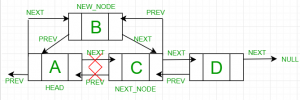Below is the implementation of the above approach:

## C++

 `// A complete working C program to demonstrate all``// insertion before a given node``#include ``#include ` `// A linked list node``struct` `Node {``    ``int` `data;``    ``struct` `Node* next;``    ``struct` `Node* prev;``};` `/* Given a reference (pointer to pointer) to the head of a``list and an int, inserts a new node on the front of the``list. */``void` `push(``struct` `Node** head_ref, ``int` `new_data)``{``    ``struct` `Node* new_node``        ``= (``struct` `Node*)``malloc``(``sizeof``(``struct` `Node));` `    ``new_node->data = new_data;` `    ``new_node->next = (*head_ref);``    ``new_node->prev = NULL;` `    ``if` `((*head_ref) != NULL)``        ``(*head_ref)->prev = new_node;` `    ``(*head_ref) = new_node;``}` `/* Given a node as next_node, insert a new node before the`` ``* given node */``void` `insertBefore(``struct` `Node** head_ref,``                  ``struct` `Node* next_node, ``int` `new_data)``{``    ``/*1. check if the given next_node is NULL */``    ``if` `(next_node == NULL) {``        ``printf``(``"the given next node cannot be NULL"``);``        ``return``;``    ``}` `    ``/* 2. allocate new node */``    ``struct` `Node* new_node``        ``= (``struct` `Node*)``malloc``(``sizeof``(``struct` `Node));` `    ``/* 3. put in the data */``    ``new_node->data = new_data;` `    ``/* 4. Make prev of new node as prev of next_node */``    ``new_node->prev = next_node->prev;` `    ``/* 5. Make the prev of next_node as new_node */``    ``next_node->prev = new_node;` `    ``/* 6. Make next_node as next of new_node */``    ``new_node->next = next_node;` `    ``/* 7. Change next of new_node's previous node */``    ``if` `(new_node->prev != NULL)``        ``new_node->prev->next = new_node;``    ``/* 8. If the prev of new_node is NULL, it will be``       ``the new head node */``    ``else``        ``(*head_ref) = new_node;``}` `// This function prints contents of linked list starting``// from the given node``void` `printList(``struct` `Node* node)``{``    ``struct` `Node* last;``    ``printf``(``"\nTraversal in forward direction \n"``);``    ``while` `(node != NULL) {``        ``printf``(``" %d "``, node->data);``        ``last = node;``        ``node = node->next;``    ``}` `    ``printf``(``"\nTraversal in reverse direction \n"``);``    ``while` `(last != NULL) {``        ``printf``(``" %d "``, last->data);``        ``last = last->prev;``    ``}``}` `/* Driver program to test above functions*/``int` `main()``{``    ``/* Start with the empty list */``    ``struct` `Node* head = NULL;``    ``push(&head, 7);` `    ``push(&head, 1);` `    ``push(&head, 4);` `    ``// Insert 8, before 1. So linked list becomes``    ``// 4->8->1->7->NULL``    ``insertBefore(&head, head->next, 8);` `    ``printf``(``"Created DLL is: "``);``    ``printList(head);` `    ``getchar``();``    ``return` `0;``}`

## Python3

 `# A complete working Python program to demonstrate all``# insertion before a given node` `# A linked list node``class` `Node:``    ``def` `__init__(``self``, x):``        ``self``.data ``=` `x``        ``self``.prev ``=` `None``        ``self``.``next` `=` `None` `# /* Given a reference (pointer to pointer)``# to the head of a list``# and an int, inserts a new node on``# the front of the list. */``def` `push(head_ref, new_data):` `    ``# /* 1. allocate node */``    ``new_node ``=` `Node(new_data)` `    ``# /* 2. put in the data */``    ``new_node.data ``=` `new_data` `    ``# /* 3. Make next of new node as``    ``# head and previous as None */``    ``new_node.``next` `=` `head_ref``    ``new_node.prev ``=` `None` `    ``# /* 4. change prev of head node to new node */``    ``if` `(head_ref !``=` `None``):``        ``head_ref.prev ``=` `new_node` `    ``# /* 5. move the head to point to the new node */``    ``head_ref ``=` `new_node` `    ``return` `head_ref` `# /* Given a node as next_node, insert``# a new node before the given node */`  `def` `insertBefore(head_ref, next_node, new_data):` `    ``# /*1. check if the given next_node is NULL */``    ``if` `(next_node ``=``=` `None``):``        ``print``(``"the given next node cannot be NULL"``)``        ``return` `    ``# /* 3. put in the data */``    ``new_node ``=` `Node(new_data)` `    ``# /* 4. Make prev of new node as prev of next_node */``    ``new_node.prev ``=` `next_node.prev` `    ``# /* 5. Make the prev of next_node as new_node */``    ``next_node.prev ``=` `new_node` `    ``# /* 6. Make next_node as next of new_node */``    ``new_node.``next` `=` `next_node` `    ``# /* 7. Change next of new_node's previous node */``    ``if` `(new_node.prev !``=` `None``):``        ``new_node.prev.``next` `=` `new_node` `    ``# /* 8. If the prev of new_node is NULL, it will be``    ``#   the new head node */``    ``else``:``        ``head_ref ``=` `new_node` `    ``return` `head_ref` `# This function prints contents of linked``# list starting from the given node``def` `printList(node):``    ``last ``=` `None``    ``print``(``"Traversal in forward direction "``)``    ``while` `(node !``=` `None``):``        ``print``(node.data, end``=``" "``)``        ``last ``=` `node``        ``node ``=` `node.``next` `    ``print``(``"\nTraversal in reverse direction "``)``    ``while` `(last !``=` `None``):``        ``print``(last.data, end``=``" "``)``        ``last ``=` `last.prev`  `# /* Driver program to test above functions*/``if` `__name__ ``=``=` `'__main__'``:` `    ``# /* Start with the empty list */``    ``head ``=` `None``    ``head ``=` `push(head, ``7``)` `    ``head ``=` `push(head, ``1``)` `    ``head ``=` `push(head, ``4``)` `    ``# Insert 8, before 1. So linked list becomes 4.8.1.7.NULL``    ``head ``=` `insertBefore(head, head.``next``, ``8``)` `    ``print``(``"Created DLL is: "``)``    ``printList(head)` `# This code is contributed by mohit kumar 29`

Output:

```Created DLL is:
Traversal in forward direction
4  8  1  7
Traversal in reverse direction
7  1  8  4```

A complete working program to test above functions.
Following is complete program to test above functions.

## C++

 `// A complete working C++ program to``// demonstrate all insertion methods``#include ``using` `namespace` `std;` `// A linked list node``class` `Node``{``    ``public``:``    ``int` `data;``    ``Node* next;``    ``Node* prev;``};` `/* Given a reference (pointer to pointer)``to the head of a list``and an int, inserts a new node on the``front of the list. */``void` `push(Node** head_ref, ``int` `new_data)``{``    ``/* 1. allocate node */``    ``Node* new_node = ``new` `Node();` `    ``/* 2. put in the data */``    ``new_node->data = new_data;` `    ``/* 3. Make next of new node as head``    ``and previous as NULL */``    ``new_node->next = (*head_ref);``    ``new_node->prev = NULL;` `    ``/* 4. change prev of head node to new node */``    ``if` `((*head_ref) != NULL)``        ``(*head_ref)->prev = new_node;` `    ``/* 5. move the head to point to the new node */``    ``(*head_ref) = new_node;``}` `/* Given a node as prev_node, insert``a new node after the given node */``void` `insertAfter(Node* prev_node, ``int` `new_data)``{``    ``/*1. check if the given prev_node is NULL */``    ``if` `(prev_node == NULL)``    ``{``        ``cout<<``"the given previous node cannot be NULL"``;``        ``return``;``    ``}` `    ``/* 2. allocate new node */``    ``Node* new_node = ``new` `Node();` `    ``/* 3. put in the data */``    ``new_node->data = new_data;` `    ``/* 4. Make next of new node as next of prev_node */``    ``new_node->next = prev_node->next;` `    ``/* 5. Make the next of prev_node as new_node */``    ``prev_node->next = new_node;` `    ``/* 6. Make prev_node as previous of new_node */``    ``new_node->prev = prev_node;` `    ``/* 7. Change previous of new_node's next node */``    ``if` `(new_node->next != NULL)``        ``new_node->next->prev = new_node;``}` `/* Given a reference (pointer to pointer) to the head``of a DLL and an int, appends a new node at the end */``void` `append(Node** head_ref, ``int` `new_data)``{``    ``/* 1. allocate node */``    ``Node* new_node = ``new` `Node();` `    ``Node* last = *head_ref; ``/* used in step 5*/` `    ``/* 2. put in the data */``    ``new_node->data = new_data;` `    ``/* 3. This new node is going to be the last node, so``        ``make next of it as NULL*/``    ``new_node->next = NULL;` `    ``/* 4. If the Linked List is empty, then make the new``        ``node as head */``    ``if` `(*head_ref == NULL)``    ``{``        ``new_node->prev = NULL;``        ``*head_ref = new_node;``        ``return``;``    ``}` `    ``/* 5. Else traverse till the last node */``    ``while` `(last->next != NULL)``        ``last = last->next;` `    ``/* 6. Change the next of last node */``    ``last->next = new_node;` `    ``/* 7. Make last node as previous of new node */``    ``new_node->prev = last;` `    ``return``;``}` `// This function prints contents of``// linked list starting from the given node``void` `printList(Node* node)``{``    ``Node* last;``    ``cout<<``"\nTraversal in forward direction \n"``;``    ``while` `(node != NULL)``    ``{``        ``cout<<``" "``<data<<``" "``;``        ``last = node;``        ``node = node->next;``    ``}` `    ``cout<<``"\nTraversal in reverse direction \n"``;``    ``while` `(last != NULL)``    ``{``        ``cout<<``" "``<data<<``" "``;``        ``last = last->prev;``    ``}``}` `/* Driver program to test above functions*/``int` `main()``{``    ``/* Start with the empty list */``    ``Node* head = NULL;` `    ``// Insert 6. So linked list becomes 6->NULL``    ``append(&head, 6);` `    ``// Insert 7 at the beginning. So``    ``// linked list becomes 7->6->NULL``    ``push(&head, 7);` `    ``// Insert 1 at the beginning. So``    ``// linked list becomes 1->7->6->NULL``    ``push(&head, 1);` `    ``// Insert 4 at the end. So linked``    ``// list becomes 1->7->6->4->NULL``    ``append(&head, 4);` `    ``// Insert 8, after 7. So linked``    ``// list becomes 1->7->8->6->4->NULL``    ``insertAfter(head->next, 8);` `    ``cout << ``"Created DLL is: "``;``    ``printList(head);` `    ``return` `0;``}` `// This is code is contributed by rathbhupendra`

## C

 `// A complete working C program to``// demonstrate all insertion``// methods``#include ``#include ` `// A linked list node``struct` `Node {``    ``int` `data;``    ``struct` `Node* next;``    ``struct` `Node* prev;``};` `/* Given a reference (pointer to pointer) to the head of a``   ``list and an int, inserts a new node on the front of the``   ``list. */``void` `push(``struct` `Node** head_ref, ``int` `new_data)``{``    ``/* 1. allocate node */``    ``struct` `Node* new_node``        ``= (``struct` `Node*)``malloc``(``sizeof``(``struct` `Node));` `    ``/* 2. put in the data  */``    ``new_node->data = new_data;` `    ``/* 3. Make next of new node as head and previous as NULL``     ``*/``    ``new_node->next = (*head_ref);``    ``new_node->prev = NULL;` `    ``/* 4. change prev of head node to new node */``    ``if` `((*head_ref) != NULL)``        ``(*head_ref)->prev = new_node;` `    ``/* 5. move the head to point to the new node */``    ``(*head_ref) = new_node;``}` `/* Given a node as prev_node, insert a new node after the`` ``* given node */``void` `insertAfter(``struct` `Node* prev_node, ``int` `new_data)``{``    ``/*1. check if the given prev_node is NULL */``    ``if` `(prev_node == NULL) {``        ``printf``(``"the given previous node cannot be NULL"``);``        ``return``;``    ``}` `    ``/* 2. allocate new node */``    ``struct` `Node* new_node``        ``= (``struct` `Node*)``malloc``(``sizeof``(``struct` `Node));` `    ``/* 3. put in the data  */``    ``new_node->data = new_data;` `    ``/* 4. Make next of new node as next of prev_node */``    ``new_node->next = prev_node->next;` `    ``/* 5. Make the next of prev_node as new_node */``    ``prev_node->next = new_node;` `    ``/* 6. Make prev_node as previous of new_node */``    ``new_node->prev = prev_node;` `    ``/* 7. Change previous of new_node's next node */``    ``if` `(new_node->next != NULL)``        ``new_node->next->prev = new_node;``}` `/* Given a reference (pointer to pointer) to the head``   ``of a DLL and an int, appends a new node at the end  */``void` `append(``struct` `Node** head_ref, ``int` `new_data)``{``    ``/* 1. allocate node */``    ``struct` `Node* new_node``        ``= (``struct` `Node*)``malloc``(``sizeof``(``struct` `Node));` `    ``struct` `Node* last = *head_ref; ``/* used in step 5*/` `    ``/* 2. put in the data  */``    ``new_node->data = new_data;` `    ``/* 3. This new node is going to be the last node, so``          ``make next of it as NULL*/``    ``new_node->next = NULL;` `    ``/* 4. If the Linked List is empty, then make the new``          ``node as head */``    ``if` `(*head_ref == NULL) {``        ``new_node->prev = NULL;``        ``*head_ref = new_node;``        ``return``;``    ``}` `    ``/* 5. Else traverse till the last node */``    ``while` `(last->next != NULL)``        ``last = last->next;` `    ``/* 6. Change the next of last node */``    ``last->next = new_node;` `    ``/* 7. Make last node as previous of new node */``    ``new_node->prev = last;` `    ``return``;``}` `// This function prints contents of linked list starting``// from the given node``void` `printList(``struct` `Node* node)``{``    ``struct` `Node* last;``    ``printf``(``"\nTraversal in forward direction \n"``);``    ``while` `(node != NULL) {``        ``printf``(``" %d "``, node->data);``        ``last = node;``        ``node = node->next;``    ``}` `    ``printf``(``"\nTraversal in reverse direction \n"``);``    ``while` `(last != NULL) {``        ``printf``(``" %d "``, last->data);``        ``last = last->prev;``    ``}``}` `/* Driver program to test above functions*/``int` `main()``{``    ``/* Start with the empty list */``    ``struct` `Node* head = NULL;` `    ``// Insert 6.  So linked list becomes 6->NULL``    ``append(&head, 6);` `    ``// Insert 7 at the beginning. So linked list becomes``    ``// 7->6->NULL``    ``push(&head, 7);` `    ``// Insert 1 at the beginning. So linked list becomes``    ``// 1->7->6->NULL``    ``push(&head, 1);` `    ``// Insert 4 at the end. So linked list becomes``    ``// 1->7->6->4->NULL``    ``append(&head, 4);` `    ``// Insert 8, after 7. So linked list becomes``    ``// 1->7->8->6->4->NULL``    ``insertAfter(head->next, 8);` `    ``printf``(``"Created DLL is: "``);``    ``printList(head);` `    ``getchar``();``    ``return` `0;``}`

## Java

 `// A complete working Java program to demonstrate all` `// Class for Doubly Linked List``public` `class` `DLL {``    ``Node head; ``// head of list` `    ``/* Doubly Linked list Node*/``    ``class` `Node {``        ``int` `data;``        ``Node prev;``        ``Node next;` `        ``// Constructor to create a new node``        ``// next and prev is by default initialized as null``        ``Node(``int` `d) { data = d; }``    ``}` `    ``// Adding a node at the front of the list``    ``public` `void` `push(``int` `new_data)``    ``{``        ``/* 1. allocate node``        ``* 2. put in the data */``        ``Node new_Node = ``new` `Node(new_data);` `        ``/* 3. Make next of new node as head and previous as NULL */``        ``new_Node.next = head;``        ``new_Node.prev = ``null``;` `        ``/* 4. change prev of head node to new node */``        ``if` `(head != ``null``)``            ``head.prev = new_Node;` `        ``/* 5. move the head to point to the new node */``        ``head = new_Node;``    ``}` `    ``/* Given a node as prev_node, insert``    ``a new node after the given node */``    ``public` `void` `InsertAfter(Node prev_Node, ``int` `new_data)``    ``{` `        ``/*1. check if the given prev_node is NULL */``        ``if` `(prev_Node == ``null``) {``            ``System.out.println(``"The given previous node cannot be NULL "``);``            ``return``;``        ``}` `        ``/* 2. allocate node``        ``* 3. put in the data */``        ``Node new_node = ``new` `Node(new_data);` `        ``/* 4. Make next of new node as next of prev_node */``        ``new_node.next = prev_Node.next;` `        ``/* 5. Make the next of prev_node as new_node */``        ``prev_Node.next = new_node;` `        ``/* 6. Make prev_node as previous of new_node */``        ``new_node.prev = prev_Node;` `        ``/* 7. Change previous of new_node's next node */``        ``if` `(new_node.next != ``null``)``            ``new_node.next.prev = new_node;``    ``}` `    ``// Add a node at the end of the list``    ``void` `append(``int` `new_data)``    ``{``        ``/* 1. allocate node``        ``* 2. put in the data */``        ``Node new_node = ``new` `Node(new_data);` `        ``Node last = head; ``/* used in step 5*/` `        ``/* 3. This new node is going to be the last node, so``        ``* make next of it as NULL*/``        ``new_node.next = ``null``;` `        ``/* 4. If the Linked List is empty, then make the new``        ``* node as head */``        ``if` `(head == ``null``) {``            ``new_node.prev = ``null``;``            ``head = new_node;``            ``return``;``        ``}` `        ``/* 5. Else traverse till the last node */``        ``while` `(last.next != ``null``)``            ``last = last.next;` `        ``/* 6. Change the next of last node */``        ``last.next = new_node;` `        ``/* 7. Make last node as previous of new node */``        ``new_node.prev = last;``    ``}` `    ``// This function prints contents of``    ``// linked list starting from the given node``    ``public` `void` `printlist(Node node)``    ``{``        ``Node last = ``null``;``        ``System.out.println(``"Traversal in forward Direction"``);``        ``while` `(node != ``null``) {``            ``System.out.print(node.data + ``" "``);``            ``last = node;``            ``node = node.next;``        ``}``        ``System.out.println();``        ``System.out.println(``"Traversal in reverse direction"``);``        ``while` `(last != ``null``) {``            ``System.out.print(last.data + ``" "``);``            ``last = last.prev;``        ``}``    ``}` `    ``/* Driver program to test above functions*/``    ``public` `static` `void` `main(String[] args)``    ``{``        ``/* Start with the empty list */``        ``DLL dll = ``new` `DLL();` `        ``// Insert 6. So linked list becomes 6->NULL``        ``dll.append(``6``);` `        ``// Insert 7 at the beginning. So``        ``.. linked list becomes ``7``->``6``->NULL``        ``dll.push(``7``);` `        ``// Insert 1 at the beginning. So``        ``// linked list becomes 1->7->6->NULL``        ``dll.push(``1``);` `        ``// Insert 4 at the end. So linked``        ``// list becomes 1->7->6->4->NULL``        ``dll.append(``4``);` `        ``// Insert 8, after 7. So linked``        ``// list becomes 1->7->8->6->4->NULL``        ``dll.InsertAfter(dll.head.next, ``8``);` `        ``System.out.println(``"Created DLL is: "``);``        ``dll.printlist(dll.head);``    ``}``}` `// This code is contributed by Sumit Ghosh`

## Python

 `# A complete working Python``# program to demonstrate all``# insertion methods` `# A linked list node``class` `Node:` `    ``# Constructor to create a new node``    ``def` `__init__(``self``, data):``        ``self``.data ``=` `data``        ``self``.``next` `=` `None``        ``self``.prev ``=` `None` `# Class to create a Doubly Linked List``class` `DoublyLinkedList:` `    ``# Constructor for empty Doubly Linked List``    ``def` `__init__(``self``):``        ``self``.head ``=` `None` `    ``# Given a reference to the head of a list and an``    ``# integer, inserts a new node on the front of list``    ``def` `push(``self``, new_data):` `        ``# 1. Allocates node``        ``# 2. Put the data in it``        ``new_node ``=` `Node(new_data)` `        ``# 3. Make next of new node as head and``        ``# previous as None (already None)``        ``new_node.``next` `=` `self``.head` `        ``# 4. change prev of head node to new_node``        ``if` `self``.head ``is` `not` `None``:``            ``self``.head.prev ``=` `new_node` `        ``# 5. move the head to point to the new node``        ``self``.head ``=` `new_node` `    ``# Given a node as prev_node, insert a new node after``    ``# the given node``    ``def` `insertAfter(``self``, prev_node, new_data):` `        ``# 1. Check if the given prev_node is None``        ``if` `prev_node ``is` `None``:``            ``print` `"the given previous node cannot be NULL"``            ``return` `        ``# 2. allocate new node``        ``# 3. put in the data``        ``new_node ``=` `Node(new_data)` `        ``# 4. Make net of new node as next of prev node``        ``new_node.``next` `=` `prev_node.``next` `        ``# 5. Make prev_node as previous of new_node``        ``prev_node.``next` `=` `new_node` `        ``# 6. Make prev_node ass previous of new_node``        ``new_node.prev ``=` `prev_node` `        ``# 7. Change previous of new_nodes's next node``        ``if` `new_node.``next``:``            ``new_node.``next``.prev ``=` `new_node` `    ``# Given a reference to the head of DLL and integer,``    ``# appends a new node at the end``    ``def` `append(``self``, new_data):` `        ``# 1. Allocates node``        ``# 2. Put in the data``        ``new_node ``=` `Node(new_data)` `        ``# 3. This new node is going to be the last node,``        ``# so make next of it as None``        ``# (It already is initialized as None)` `        ``# 4. If the Linked List is empty, then make the``        ``# new node as head``        ``if` `self``.head ``is` `None``:``            ``self``.head ``=` `new_node``            ``return` `        ``# 5. Else traverse till the last node``        ``last ``=` `self``.head``        ``while` `last.``next``:``            ``last ``=` `last.``next` `        ``# 6. Change the next of last node``        ``last.``next` `=` `new_node` `        ``# 7. Make last node as previous of new node``        ``new_node.prev ``=` `last` `        ``return` `    ``# This function prints contents of linked list``    ``# starting from the given node``    ``def` `printList(``self``, node):` `        ``print` `"\nTraversal in forward direction"``        ``while` `node:``            ``print` `" % d"` `%` `(node.data),``            ``last ``=` `node``            ``node ``=` `node.``next` `        ``print` `"\nTraversal in reverse direction"``        ``while` `last:``            ``print` `" % d"` `%` `(last.data),``            ``last ``=` `last.prev` `# Driver program to test above functions`  `# Start with empty list``llist ``=` `DoublyLinkedList()` `# Insert 6. So the list becomes 6->None``llist.append(``6``)` `# Insert 7 at the beginning.``# So linked list becomes 7->6->None``llist.push(``7``)` `# Insert 1 at the beginning.``# So linked list becomes 1->7->6->None``llist.push(``1``)` `# Insert 4 at the end.``# So linked list becomes 1->7->6->4->None``llist.append(``4``)` `# Insert 8, after 7.``# So linked list becomes 1->7->8->6->4->None``llist.insertAfter(llist.head.``next``, ``8``)` `print` `"Created DLL is: "``,``llist.printList(llist.head)` `# This code is contributed by Nikhil Kumar Singh(nickzuck_007)`

## C#

 `// A complete working C# program to demonstrate all``using` `System;` `// Class for Doubly Linked List``public` `class` `DLL``{``    ``Node head; ``// head of list` `    ``/* Doubly Linked list Node*/``    ``public` `class` `Node``    ``{``        ``public` `int` `data;``        ``public` `Node prev;``        ``public` `Node next;` `        ``// Constructor to create a new node``        ``// next and prev is by default initialized as null``        ``public` `Node(``int` `d)``        ``{``            ``data = d;``        ``}``    ``}` `    ``// Adding a node at the front of the list``    ``public` `void` `push(``int` `new_data)``    ``{``        ``/* 1. allocate node``        ``* 2. put in the data */``        ``Node new_Node = ``new` `Node(new_data);` `        ``/* 3. Make next of new node as``        ``head and previous as NULL */``        ``new_Node.next = head;``        ``new_Node.prev = ``null``;` `        ``/* 4. change prev of head node to new node */``        ``if` `(head != ``null``)``            ``head.prev = new_Node;` `        ``/* 5. move the head to point to the new node */``        ``head = new_Node;``    ``}` `    ``/* Given a node as prev_node, insert``    ``a new node after the given node */``    ``public` `void` `InsertAfter(Node prev_Node, ``int` `new_data)``    ``{` `        ``/*1. check if the given prev_node is NULL */``        ``if` `(prev_Node == ``null``)``        ``{``            ``Console.WriteLine(``"The given previous node cannot be NULL "``);``            ``return``;``        ``}` `        ``/* 2. allocate node``        ``* 3. put in the data */``        ``Node new_node = ``new` `Node(new_data);` `        ``/* 4. Make next of new node as next of prev_node */``        ``new_node.next = prev_Node.next;` `        ``/* 5. Make the next of prev_node as new_node */``        ``prev_Node.next = new_node;` `        ``/* 6. Make prev_node as previous of new_node */``        ``new_node.prev = prev_Node;` `        ``/* 7. Change previous of new_node's next node */``        ``if` `(new_node.next != ``null``)``            ``new_node.next.prev = new_node;``    ``}` `    ``// Add a node at the end of the list``    ``void` `append(``int` `new_data)``    ``{``        ``/* 1. allocate node``        ``* 2. put in the data */``        ``Node new_node = ``new` `Node(new_data);` `        ``Node last = head; ``/* used in step 5*/` `        ``/* 3. This new node is going``            ``to be the last node, so``        ``* make next of it as NULL*/``        ``new_node.next = ``null``;` `        ``/* 4. If the Linked List is empty,``        ``then make the new * node as head */``        ``if` `(head == ``null``)``        ``{``            ``new_node.prev = ``null``;``            ``head = new_node;``            ``return``;``        ``}` `        ``/* 5. Else traverse till the last node */``        ``while` `(last.next != ``null``)``            ``last = last.next;` `        ``/* 6. Change the next of last node */``        ``last.next = new_node;` `        ``/* 7. Make last node as previous of new node */``        ``new_node.prev = last;``    ``}` `    ``// This function prints contents of``    ``// linked list starting from the given node``    ``public` `void` `printlist(Node node)``    ``{``        ``Node last = ``null``;``        ``Console.WriteLine(``"Traversal in forward Direction"``);``        ``while` `(node != ``null``) {``            ``Console.Write(node.data + ``" "``);``            ``last = node;``            ``node = node.next;``        ``}``        ``Console.WriteLine();``        ``Console.WriteLine(``"Traversal in reverse direction"``);``        ``while` `(last != ``null``) {``            ``Console.Write(last.data + ``" "``);``            ``last = last.prev;``        ``}``    ``}` `    ``/* Driver code*/``    ``public` `static` `void` `Main(String[] args)``    ``{``        ``/* Start with the empty list */``        ``DLL dll = ``new` `DLL();` `        ``// Insert 6. So linked list becomes 6->NULL``        ``dll.append(6);` `        ``// Insert 7 at the beginning.``        ``// So linked list becomes 7->6->NULL``        ``dll.push(7);` `        ``// Insert 1 at the beginning.``        ``// So linked list becomes 1->7->6->NULL``        ``dll.push(1);` `        ``// Insert 4 at the end. So linked list``        ``// becomes 1->7->6->4->NULL``        ``dll.append(4);` `        ``// Insert 8, after 7. So linked list``        ``// becomes 1->7->8->6->4->NULL``        ``dll.InsertAfter(dll.head.next, 8);` `        ``Console.WriteLine(``"Created DLL is: "``);``        ``dll.printlist(dll.head);``    ``}``}` `// This code is contributed by 29AjayKumar`

Output:

``` Created DLL is:
Traversal in forward direction
1  7  8  6  4
Traversal in reverse direction
4  6  8  7  1```

Also see: Delete a node in double Link List
Please write comments if you find anything incorrect, or you want to share more information about the topic discussed above.

Attention reader! Don’t stop learning now. Get hold of all the important DSA concepts with the DSA Self Paced Course at a student-friendly price and become industry ready.  To complete your preparation from learning a language to DS Algo and many more,  please refer Complete Interview Preparation Course.

My Personal Notes arrow_drop_up